# Face-off with Sizeof()

0
6047In C programming, the unary operator sizeof() returns the size of its operand in bytes. The sizeof() operator is discussed in detail in this article, along with illustrative examples of code.

Sizeof() is used extensively in the C programming language. It is a compile-time unary operator, which can be used to compute the size (in bytes) of any data type of its operands. The operand may be an actual type-specifier (such as char or ints) or any valid expression. The sizeof() operator returns the total memory allocated for a particular object in terms of bytes. The resultant type of the sizeof() operator is size_t. Sizeof() can be applied both for primitive data types (such as char, ints, floats, etc) including pointers and also for the compound data types (such as structures, unions, etc).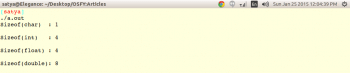Figure 1: Output of the demo program shown in Code 1

Usage
The sizeof() operator can be used in two different cases depending upon the operand types. Let me elaborate this with syntax, examples and sample codes.

Case 1: When the operand is a type-name
When sizeof() is used with type-name as the operand (such as char, int, float, double, etc), it returns the amount of memory that will be used by an object of that type. In this case, the operand should be enclosed within parenthesis. The sizeof operator is used when the operand is a type-name as shown in Table 1.
Illustration: The sample demo code is shown below (Code 1), and the output of the code when I run it in my system is shown in Figure 1.

Code 1

```1 #include <stdio.h>
2 #include <stddef.h>
3
4 int main()
5 {
6 printf(Sizeof(char) : %lu\n, (long unsigned ) sizeof(char));
7 printf(Sizeof(int) : %lu\n, (long unsigned ) sizeof(int));
8 printf(Sizeof(float) : %lu\n, (long unsigned ) sizeof(float));
9 printf(Sizeof(double) : %lu\n, (long unsigned ) sizeof(double));
10 return 0;
11 }```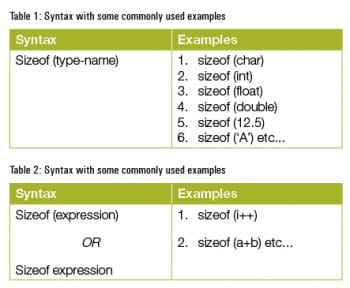Note 1: The return value of the sizeof() operator is implementation-defined, and its type (an unsigned integer type) is size_t, defined in <stddef.h>.

Note 2: C99 has included %zu as a type specifier
for size_t. But for older compilers, %z will fail. Hence, use %lu or %llu along with a typecasting to achieve portability of the code across various platforms.

Case 2: When the operand is an expression
When sizeof() is used with expression as an operand, the operand can be enclosed with or without parenthesis. The syntax of how the sizeof() operator is used preceding the expression is shown in Table 2 along with some examples.
Illustration: The sample demo code is shown in the Code 2 snippet, and the output of the code when I run it in my system is shown in Figure 2.

Code 2

```1 #include <stdio.h>
2 #include <stddef.h>
3
4 int main()
5 {
6 int a = 10;
7
8 double d = 12.34;
9
10 printf(Sizeof( a + d ): %lu\n, (long unsigned) sizeof(a + d));
11
12 return 0;
13 }```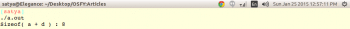Figure 2: Output of the demo program shown in Code 2

From the above demo code, it is very clear that when the sizeof() operator is applied to an expression, it yields a result that is the same as if it had been applied to the type-name of the resultant of the expression. Since, at compile time the compiler analyses the expression to determine its type, but it will never evaluate the expression which takes place at runtime.
In the example shown in Code 2, a is of int type andd is of double type. When type conversion is applied, as usual, the lower rank data type is promoted to a higher rank data type and the resultant data type is nothing but a double in our case; hence, sizeof( a + d ) yields sizeof(double) which is 8 bytes as shown in Figure 2. In general, if the operand contains the operators that perform type conversions, the compiler considers these conversions in determining the type of the expression.
Behaviour
The sizeof() operator behaves differently in comparison with other operators. In this article, let me point out the uniqueness of this operator by taking two real-time programming examples. The first is about compile-time behaviour and the second one is about runtime behaviour.
Case 1: Compile-time behaviour
To start with, let us consider the simple code as shown in the Code 3 snippet.
Code 3

```1 #include <stdio.h>
2 #include <stddef.h>
3
4 int main()
5 {
6 int i = 10;
7
8 size_t size = sizeof(i++);
9
10 printf(Size of i : %lu\n, (long unsigned ) size);
11
12 printf(value of i : %d\n, i);
13
14 return 0;
15 }```

Can you guess what the output of the above mentioned program will be? At first glance, anybody would say it is 4 (assuming the sizeof(int) is 4 bytes) and 11. But, when I run the program in my system, it shows 4 and 10 (refer Figure 3 for output).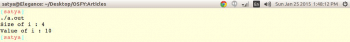Figure 3: Output of the demo program shown in code 3

Why are we getting the value of variable i as 10 instead of 11? Here is the reason.
The sizeof operator is the only one in C, which is evaluated at compile time, where sizeof(i++) in our example is replaced by the value 4 during compile time itself. We can validate this by referring to Figure 4, which contains the assembly code equivalent to the sizeof(i++) in C.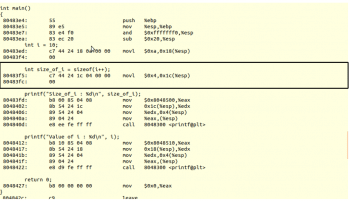Figure 4: Assembly code generated by the compiler for code 3.

Note: To obtain the assembly code as shown in Figure 4, follow the steps shown below:
+ gcc -g filename.c (in our case, the file name is sizeof_run.c)
+ objdump -S output_file (in our case, the output file is a.out)

From Figure 4, we can see that sizeof() is completely evaluated at compile time (the exception is gcc, which supports zero-sized structures as a GNU extension, which is evaluated at the runtime). And the whole sizeof(i++) is replaced by the constant value 4, which is highlighted in the box. Hence, there are no assembly instructions for i++ at all, which is supposed to be evaluated at the runtime.

Case 2: Runtime behaviour
As mentioned earlier, sizeof() is the only operator in C, which is evaluated at the compile time. But, there is an exception for this in C99 standards, for variable length arrays.
To start with, let us consider the following code (Code 4):

Code 4

```1 #include <stdio.h>
2 #include <stddef.h>
3
4 int main()
5 {
6 unsigned int size;
7 size_t array_size;
8
9 printf(Enter the size:);
10 scanf(%u, &size);
11
12 //Declaring the variable length array
13 int array[size];
14
15 //Finding the size of array
16 array_size = sizeof(array);
17
18 printf(Size of array : %lu\n, (long unsigned ) array_size);
19
20 return 0;
21 }```

Let us see the output, when the above code is compiled and run (shown in Figure 5).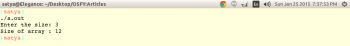Figure 5: Output of the sizeof_run.c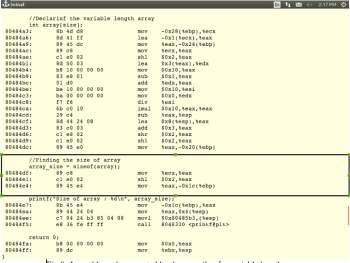Figure 6: Assembly code generated by the compiler, for variable length array

From the above output it is very clear that the sizeof() operator is evaluated at runtime. We can observe the equivalent assembly code generated by the compiler as shown in Figure 6.
Also, note the difference between the assembly code in Figures 4 and 6.
The need for sizeof()
Case 1: Auto determination of the number of elements in an array
To compute the number of elements of the array automatically, depending on the data-type of the element, the sizeof() operator comes in handy.
For an explanation, let us consider the code snippet given below:

```1 #include <stdio.h>
2 #include <stddef.h>
3
4 int main()
5 {
6 int array[] = {10, 20, 30, 40, 50};
7
8 size_t i;
9
10 for(i = 0; i < sizeof(array) / sizeof(array); i++)
11 {
12 //some code
13 }
14 }```

In the example shown above, at line number 10, by using the sizeof() operator the number of elements is automatically computed.
The sizes of primitive data types in C are implementation defined. For example, the sizeof(long) on 32-bit architecture may vary from that on 64-bit architecture. So, when we decide statically the sizeof(long) as 4 bytes wide on 32-bit architecture, and when the same code is ported to 64-bit architecture, the results may go wrong. So, in order to avoid the portability issue, it is a best practice to use sizeof() to compute the sizeof variables or arrays, depending on the exact size of a particular data type.

Case 2: To allocate a block of memory dynamically of a particular data type
In case of dynamic memory allocation of an array, sizeof() plays an important role. For example, if we want to allocate a block of memory that is big enough to hold 5 integers in an array, sizeof comes in handy and is a great help, since we do not know the exact sizeof(int) to dynamically allocate the memory using malloc function for a particular architecture.

`int *iptr = malloc( 5* sizeof(int));`

In the above example, we are mallocing the block of memory, which is equal to the number of bytes of type int, multiplied by 5, ensuring sufficient space for all five ints is allocated.
Case 3: To determine the sizeof compound data types
Sometimes, it is very difficult to predict the sizes of compound data types such as structures, due to structure padding, and to predict the size of unions. Sizeof() is of great use here.

Cases when sizeof() will not work
The sizeof operator will not work when applied to the following cases:

• A bit field
• A function type
• An incomplete type (such as void)
• Zero-sized array (except in GCC, which supports zero-sized structures as a GNU extension).

sizeof() and incomplete data types
An incomplete type in C is one that describes an identifier, but lacks the information needed to determine the size of the identifier.
Examples of incompletely defined types are:

1. An array type whose dimensions have not yet been specified
2. A structure type whose members have not yet been specified

Illustration: An array whose dimensions are not specified

 File1.cint array; File2.cextern int array;

In the above example, for the code in file1.c, sizeof() can be applied to find the size of the array, as it is completely defined in file1.c. But, in file2.c, the sizeof() operator will not work since the dimensions of the array are missing. Without this information, the compiler has no knowledge of how many elements are in the array and cannot calculate the sizeof of the array.

How sizeof() is different from a function call
Let us consider the following code to understand how sizeof() is different from a function call:

```1 #include <stdio.h>
2 #include <stddef.h>
3
4 int main()
5 {
6 int x = 5;
7
8 printf(%lu:%lu:%lu\n, (long unsigned )sizeof(int), (long unsigned )sizeof x, (long unsigned )sizeof 5);
9
10 return 0;
11 }```

In the above example, sizeof() will work even if the braces are not present across operands, whereas in functions, braces are a must. So, here are three reasons why sizeof is not a function:
1. It can be applied for any type of operand.
2. It can also be used, when type is an operand.
3. No brackets needed across operands
You can implement your own SIZEOF() macro, which should work like a sizeof() operator.
According to the C99 standards, the sizeof() operator yields the size (in integer bytes) of its operand, which may be an expression or the parenthesised name of a type. If the type of the operand is a variable length array type, the operand is evaluated at runtime; otherwise, the operand is not evaluated and the result is an integer constant, during the compile time itself.